Pay with
Markets
Derivatives
Earn
NFT
Institutional
Feed

# FAQAccount FunctionsTutorialBinance Fan TokenBinance EarnCrypto Deposit/Withdrawal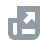Crypto Derivatives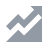FinanceAPISecurityOther Topics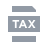Tax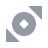Binance Convert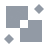NFTVIP
Home
Support Center
FAQ
Binance Cross Margin Collateral Haircuts

# Binance Cross Margin Collateral Haircuts

2023-01-05 06:40
To determine your maximum borrow and transfer-out amounts (the maximum amount that can be withdrawn from your Cross Margin account), Binance introduced the “Collateral Value”. Certain margin assets may be valued on a discounted basis using a “Collateral Ratio”, a percentage at which the asset is recognized as collateral. The Collateral Ratio is tiered, it may change as the relevant margin asset amount in your Cross Margin account increases.
Collateral Value refers to the total value of all assets in your Cross Margin account (in USDT), taking into account the relevant Collateral Ratio (the percentage at which the relevant asset is valued).
Please note that the Collateral Value will not impact your Margin Level. It will continue to be calculated based on the total asset value without any haircuts.
Different assets in your Cross Margin account have different Collateral Ratios. Please click here to view the Collateral Ratios of all eligible margin assets. Binance may, from time to time, change the Collateral Ratio at its sole discretion.
The below examples demonstrate how the tiered Collateral Ratio affects the calculation of Collateral Value.
Example 1
Scenario 1
User A holds 6,000 BTC in their Cross Margin account and the BTC price is 20,000 USDT.
The Collateral Value is calculated as follows:
USDT notional = 6,000 * 20,000 = 120,000,000 USDT
Tier 1 Collateral Ratio (USDT notional < 100,000,000) = 100%
Tier 2 Collateral Ratio (USDT notional 100,000,000 > 120,000,000) = 97.5%
Collateral Value (USDT) = (100,000,000) * 100% + (120,000,000 - 100,000,000) * 97.5% = 119,500,000 USDT
Example 2
Scenario 2
User A holds 15,000,000 USDT of Token X. The Collateral Value is calculated as follows:
USDT notional = 15,000,000
Tier 1 Collateral Ratio (USDT notional < 500,000) = 100%
Tier 2 Collateral Ratio (USDT notional 500,000 > 1,000,000) = 90%
Tier 3 Collateral Ratio (USDT notional 1,000,000 > 2,000,000) = 80%
Tier 4 Collateral Ratio (USDT notional 2,000,000 > 4,000,000) = 60%
Tier 5 Collateral Ratio (USDT notional 4,000,000 > 7,000,000) = 30%
Tier 6 Collateral Ratio (USDT notional 7,000,000 > 10,000,000) = 10%
Tier 7 Collateral Ratio (USDT notional > 10,000,000) = 0%
Collateral Value (USDT) = 500,000 * 100% + 500,000 * 90% + 1,000,000 * 80% + 2,000,000 * 60% + 3,000,000 * 30% + 3,000,000 * 10% + 5,000,000 * 0% = 4,150,000 USDT